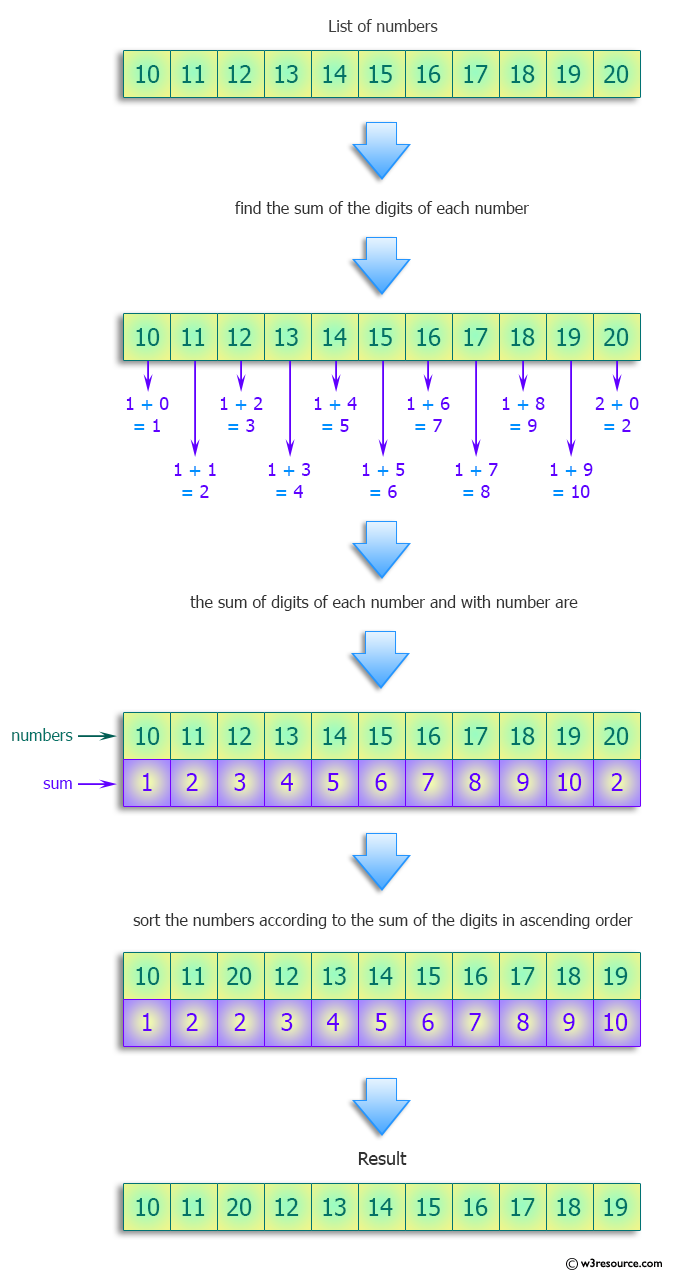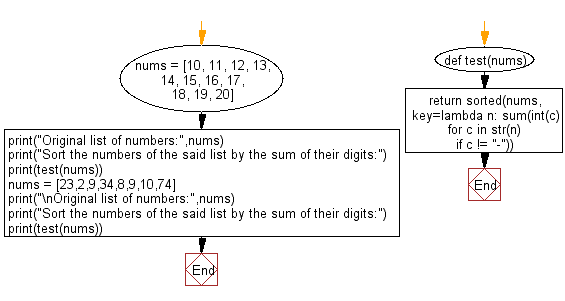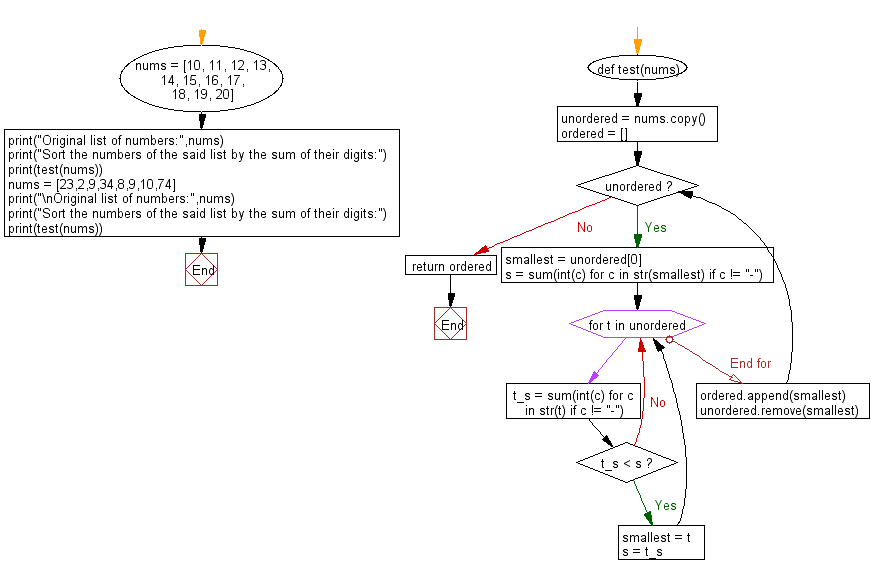﻿ Python: Sort the numbers by the sum of their digits - w3resource# Python: Sort the numbers by the sum of their digits

## Python Programming Puzzles: Exercise-38 with Solution

Write a Python program to sort the numbers of a given list by the sum of their digits.

```Input:
[10, 11, 12, 13, 14, 15, 16, 17, 18, 19, 20]
Output:
[10, 11, 20, 12, 13, 14, 15, 16, 17, 18, 19]

Input:
[23, 2, 9, 34, 8, 9, 10, 74]
Output:
[10, 2, 23, 34, 8, 9, 9, 74]
```

Pictorial Presentation:Sample Solution-1:

Python Code:

``````#License: https://bit.ly/3oLErEI

def test(nums):
return sorted(nums, key=lambda n: sum(int(c) for c in str(n) if c != "-"))

nums = [10, 11, 12, 13, 14, 15, 16, 17, 18, 19, 20]
print("Original list of numbers:",nums)
print("Sort the numbers of the said list by the sum of their digits:")
print(test(nums))
nums = [23,2,9,34,8,9,10,74]
print("\nOriginal list of numbers:",nums)
print("Sort the numbers of the said list by the sum of their digits:")
print(test(nums))
``````

Sample Output:

```Original list of numbers: [10, 11, 12, 13, 14, 15, 16, 17, 18, 19, 20]
Sort the numbers of the said list by the sum of their digits:
[10, 11, 20, 12, 13, 14, 15, 16, 17, 18, 19]

Original list of numbers: [23, 2, 9, 34, 8, 9, 10, 74]
Sort the numbers of the said list by the sum of their digits:
[10, 2, 23, 34, 8, 9, 9, 74]
```

Flowchart:## Visualize Python code execution:

The following tool visualize what the computer is doing step-by-step as it executes the said program:

Sample Solution-2:

Python Code:

``````#License: https://bit.ly/3oLErEI

def test(nums):
unordered = nums.copy()
ordered = []
while unordered:
# The number that comes first, since the list is sorted by the primes
smallest = unordered
s = sum(int(c) for c in str(smallest) if c != "-")
for t in unordered:
t_s = sum(int(c) for c in str(t) if c != "-")
if t_s < s:
smallest = t
s = t_s
ordered.append(smallest)
unordered.remove(smallest)
return ordered

nums = [10, 11, 12, 13, 14, 15, 16, 17, 18, 19, 20]
print("Original list of numbers:",nums)
print("Sort the numbers of the said list by the sum of their digits:")
print(test(nums))
nums = [23,2,9,34,8,9,10,74]
print("\nOriginal list of numbers:",nums)
print("Sort the numbers of the said list by the sum of their digits:")
print(test(nums))
``````

Sample Output:

```Original list of numbers: [10, 11, 12, 13, 14, 15, 16, 17, 18, 19, 20]
Sort the numbers of the said list by the sum of their digits:
[10, 11, 20, 12, 13, 14, 15, 16, 17, 18, 19]

Original list of numbers: [23, 2, 9, 34, 8, 9, 10, 74]
Sort the numbers of the said list by the sum of their digits:
[10, 2, 23, 34, 8, 9, 9, 74]
```

Flowchart:## Visualize Python code execution:

The following tool visualize what the computer is doing step-by-step as it executes the said program:

Python Code Editor :

Have another way to solve this solution? Contribute your code (and comments) through Disqus.

What is the difficulty level of this exercise?

Test your Programming skills with w3resource's quiz.

﻿

## Python: Tips of the Day

Clamps num within the inclusive range specified by the boundary values x and y:

Example:

```def tips_clamp_num(num,x,y):
return max(min(num, max(x, y)), min(x, y))
print(tips_clamp_num(2, 4, 6))
print(tips_clamp_num(1, -1, -6))
```

Output:

```4
-1
```